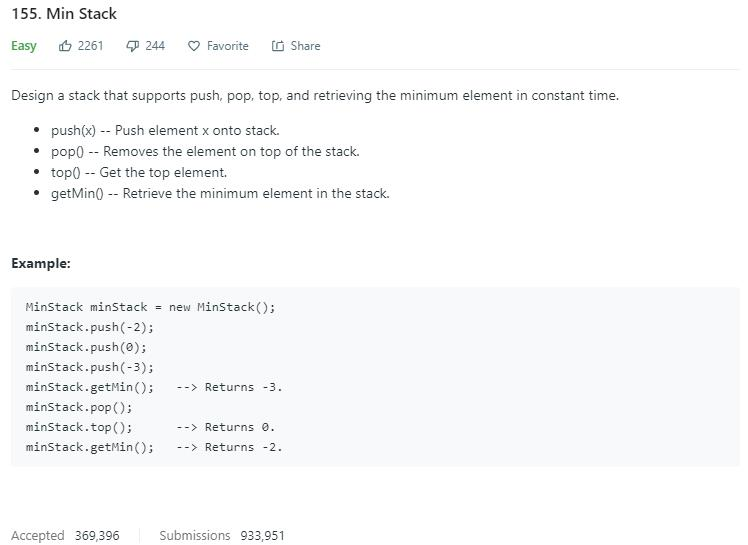# 题目描述（简单难度）# 解法一

入栈 3
|   |    |   |
|   |    |   |
|_3_|    |_3_|
stack  minStack

|   |    |   |
| 5 |    |   |
|_3_|    |_3_|
stack  minStack

| 2 |    |   |
| 5 |    | 2 |
|_3_|    |_3_|
stack  minStack

|   |    |   |
| 5 |    |   |
|_3_|    |_3_|
stack  minStack

|   |    |   |
|   |    |   |
|_3_|    |_3_|
stack  minStack

|   |    |   |
|   |    |   |
|_ _|    |_ _|
stack  minStack


class MinStack {
/** initialize your data structure here. */
private Stack<Integer> stack;
private Stack<Integer> minStack;

public MinStack() {
stack = new Stack<>();
minStack = new Stack<>();
}

public void push(int x) {
stack.push(x);
if (!minStack.isEmpty()) {
int top = minStack.peek();
//小于的时候才入栈
if (x <= top) {
minStack.push(x);
}
}else{
minStack.push(x);
}
}

public void pop() {
int pop = stack.pop();

int top = minStack.peek();
//等于的时候再出栈
if (pop == top) {
minStack.pop();
}

}

public int top() {
return stack.peek();
}

public int getMin() {
return minStack.peek();
}
}


# 解法二

入栈 3
|   |   min = 3
|   |
|_3_|
stack

|   |   min = 3
| 5 |
|_3_|
stack

| 2 |   min = 2?
| 5 |
|_3_|
stack


入栈 2 ，同时将之前的 min 值 3 入栈，再把 2 入栈，同时更新 min = 2
| 2 |   min = 2
| 3 |
| 5 |
|_3_|
stack

| 6 |  min = 2
| 2 |
| 3 |
| 5 |
|_3_|
stack

| 2 |   min = 2
| 3 |
| 5 |
|_3_|
stack

| 2 |   min = 2
| 3 |
| 5 |
|_3_|
stack


出栈 2
|   |  min = 3
| 5 |
|_3_|
stack


class MinStack {
int min = Integer.MAX_VALUE;
Stack<Integer> stack = new Stack<Integer>();
public void push(int x) {
//当前值更小
if(x <= min){
//将之前的最小值保存
stack.push(min);
//更新最小值
min=x;
}
stack.push(x);
}

public void pop() {
//如果弹出的值是最小值，那么将下一个元素更新为最小值
if(stack.pop() == min) {
min=stack.pop();
}
}

public int top() {
return stack.peek();
}

public int getMin() {
return min;
}
}


# 解法三

入栈 3，存入 3 - 3 = 0
|   |   min = 3
|   |
|_0_|
stack

|   |   min = 3
| 2 |
|_0_|
stack

| -1|   min = 2
| 5 |
|_3_|
stack

| 4 |   min = 2
| -1|
| 5 |
|_3_|
stack

|   |   min = 2
| -1|
| 5 |
|_3_|
stack

|   | min = 3
| 5 |
|_3_|
stack


public class MinStack {
long min;
Stack<Long> stack;

public MinStack(){
stack=new Stack<>();
}

public void push(int x) {
if (stack.isEmpty()) {
min = x;
stack.push(x - min);
} else {
stack.push(x - min);
if (x < min){
min = x; // 更新最小值
}

}
}

public void pop() {
if (stack.isEmpty())
return;

long pop = stack.pop();

//弹出的是负值，要更新 min
if (pop < 0) {
min = min - pop;
}

}

public int top() {
long top = stack.peek();
//负数的话，出栈的值保存在 min 中
if (top < 0) {
return (int) (min);
//出栈元素加上最小值即可
} else {
return (int) (top + min);
}
}

public int getMin() {
return (int) min;
}
}


# 解法四

class MinStack {
class Node{
int value;
int min;
Node next;

Node(int x, int min){
this.value=x;
this.min=min;
next = null;
}
}
//每次加入的节点放到头部
public void push(int x) {
}else{
//当前值和之前头结点的最小值较小的做为当前的 min
Node n = new Node(x, Math.min(x,head.min));
}
}

public void pop() {
}

public int top() {
return -1;
}

public int getMin() {